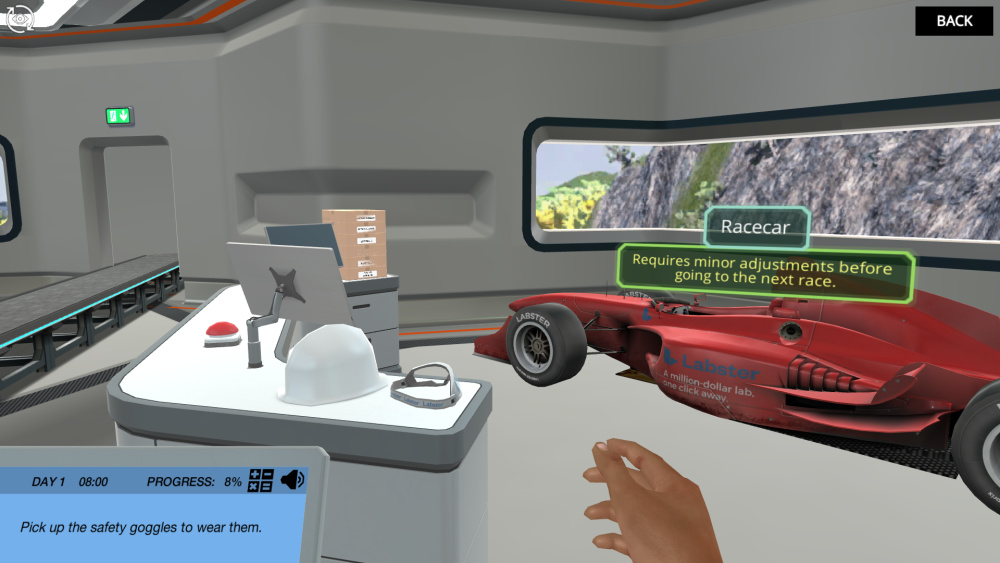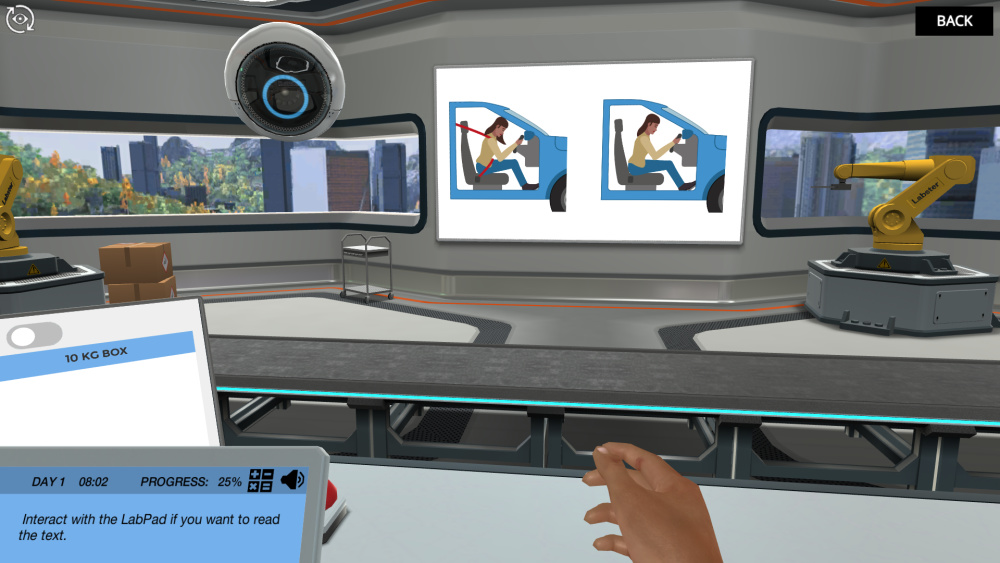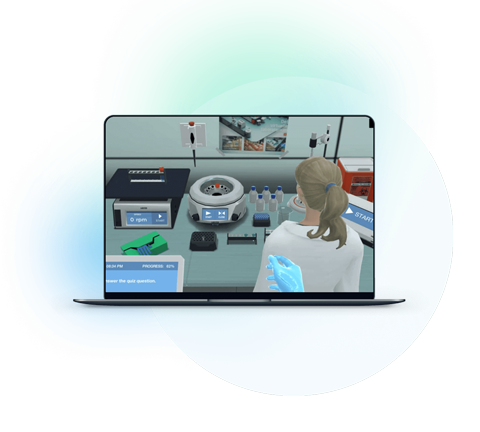Blog

# 5 Ways To Get Students Energized About Newton’s Laws

Teaching with Labster
October 11, 2022

Everything around us, whether a pencil rolling on a table or a large satellite orbiting around Earth, obeys Newton's laws of motion.

Each law explains a different aspect of motion.  The first law mentions the inertia property of matter. It states that an object remains in its current state (either rest or motion) until acted upon by an unbalanced force.

And when an unbalanced force acts on it, it starts moving; that’s the second law. This law explains the relationship between the net force, mass, and acceleration of an object. Then, there is a third that explains the action and reaction forces.

Each of these laws governs the entire field of mechanics. Every movement taking place around us follows these rules. If you are teaching an introductory physics course, you will encounter these laws. However, some students find these laws abstract and hard to understand.

Read on to learn five ways through which you can get students energized about Newton’s laws.This figure shows Newton's First Law of Motion: Balanced and unbalanced forces simulation by Labster.

## Why Newton Laws can Trickier for Students?

Physics topics usually revolve around physical processes happening around us. However, the law statements may sound confusing to students. Here are three reasons why it is so:

### 1. Abstract Forces

Multiple invisible forces act on an object at the same time. Some of the forces are easy to understand as you can see them. Just like a person pushing or pulling an object. You can easily understand the source and direction of the exerted force. But many forces like gravity, friction, and upthrust forces are invisible.

Moreover, when we talk about the concept of gravitation or force of gravity, students fail to understand the origin of these forces.

### 2. The Concept of Relativity is Confusing

Newton’s laws of motion are constrained to a frame of reference i.e., the conditions vary with the change in frame of reference. The concept of rest and motion is based on relativity.

For instance, a passenger sitting inside a bus is at rest for other passengers inside the bus with them. However, to a person standing outside on the road, the person is in motion. If we look into the bigger context, the same person standing outside the bus will be in motion to an astronaut in space since the Earth is in motion.

So, whenever we say an object is at rest or motion, that is subjective to some frame of reference. This concept of relative motion confuses a lot of students.

### 3. Requires Critical Thinking

Laws of physics, including newton’s law of motion, require critical thinking. As mentioned earlier, you have to pay attention to understand the concept of motion and rest.

The laws that Newton defined govern every motion around us. However, since students don’t observe and think critically, they fail to understand these laws.

## 5 Ways to Make Newton Laws Fun and Interesting

Since we have covered the main reasons why some students find Newton's laws tricky, let’s discuss five ways to energize students about Newton's Laws.

### 1. Show them the People Behind It

The best tool to grab students' attention is storytelling. They’re likely to listen, when you say, I am going to tell you a story. When you tell them the tales of scientists who discovered it and how they get to know these laws, the discussion becomes quite interesting.

Luckily, in this case, you have an interesting tale of the Great Isaac Newton, who proposed the three laws of motion and the law of gravity.

Isaac Newton: The Tale of Falling Apple

In the context of the law of gravitation, the tale of Falling Apple is very famous. Isaac Newton, a young boy, 23 years of age, was sitting under a tree. Suddenly, an apple fell straight on his head which led him to the concept of gravity.

Newton further said that gravity was the same force that was responsible for the planetary motion of objects in space. Later, in 1987, he published gravitation and laws of motion in the book “Principia Mathematica Philosophiae Naturalis”.

Some scientists suggest that the story is a legend. An apple may have fallen but it isn’t sure the reason Newton came up with gravity. Moreover, Newton cannot be solely credited for the laws of motion and concept. There were scientists who put forward these ideas before that.

In 1618, a German Astronomer, Johannes Kepler proposed his three laws on planetary motion. He explained the motion and orbits of planets. Then, later another astronomer, Galili Galileo was the first one to explain the inertia, motion, and velocity of objects. He was a great astronomer who put forward the idea that Earth revolves around the Sun which was unwelcomed by the Catholics at that time.

Newton was the one who used Kepler's laws and the theories of Galileo to put forward his three laws of motion. In fact, Newton’s first law is quite similar to Galileo’s law of inertia.

### 2. Explain Fundamental Concepts with Solid Examples

Always start with the basics. Do not start explaining the topic directly. Create a strong base on which the whole concept is based.

For instance, in this case, if you just start with a statement of the law, it is unlikely that students will understand. So,  start your lecture with basic terminologies used in these laws like rest, motion, inertia, force, and friction.

Rest and Motion

When discussing Newton's laws explicitly differentiate between two rest and motion. An object is said to be at rest if its position doesn’t change over time with respect to its surrounding. For instance, a tree outside your house is at rest, since its position isn’t changing in reference to your house or the neighborhood.

But if you change the frame of reference. For instance, to an astronaut in space, the object is in motion since Earth is moving with respect to the astronaut. So, both these terms (rest & motion) are subjective to a reference point. Two cars moving at the same speed are in motion to the people standing outside, however, to the riders in them, each car seems to be at rest.

Inertia

Inertia means the resistance of an object to remain in its current state. For instance, if you push a heavy table placed on the floor, at first, when resists; it doesn’t move. However, when you apply more force and overcome its inertia, it moves. The greater the mass, the greater the inertia. That is why heavy objects are difficult to move.

Similarly, a moving object doesn’t stop instantly. For instance, a car doesn’t stop instantly when you apply brakes, it travels some distance before it comes to a halt.

Motion Laws in Simple Terms

Newton’s first law says an object remains at rest or in motion until a balanced force acts on it. That is common sense. An object doesn’t move until you apply force, nor does it stop until you apply a counter force. For instance, a ball will keep rolling if there is no friction.

Newton moves a step further, in the second law. First, he says, the object doesn’t move until an unbalanced force acts on it. But as soon as a net force acts, it starts to move. In other words, if we say the object is moving, it means some kind of net force is acting on it.

When we say it’s moving, it will obviously have some velocity and acceleration. Newton’s second law says that acceleration is proportional to the net force. The more force you, the faster it will accelerate. When we eliminate that proportionality sign, a constant “mass” comes in place. So, that’s how we end up getting, F=ma.

The third law is like a cause-and-effect case. To every action there is a reaction, which is equal in magnitude to the action, however, its direction is opposite. For instance, when you jump, you apply force on the ground. In reaction, the ground applies the same amount of force and you get to jump in the car.

### 3. Assign Students Science Projects

After you have explicitly explained Newton's laws with examples, form groups of students and assign them to create a project that will demonstrate Newton's laws.

Ask them to take inspiration from the toys and objects around. This will improve their team skills and during the process, they will actually learn the practical importance of these laws.This figure shows a racecar in Newton's First Law of Motion: Balanced and unbalanced forces simulation by Labster.

### 4. Relate it to The World

Teaching the laws without their real-life application is never going to work. Since the main crux of these laws is to define the motion of objects, you must quote some really good applications.

The safety belts we use in cars are designed to prevent us from hitting the dashboard or windscreen in case of an accident. Whenever the car suddenly hits an obstruction, the passenger is forced to move outward due to inertia.

Moreover, the rockets we see in the sky, are based on Newton’s third law. The fuel gases inside the rocket burn and rush outward. As a reaction, the rocket moves forward in an upward direction.  The motion of satellites around the Earth is also based on Newton's gravitation law.

In short, from a small toy car in your house to a huge satellite around the Earth, the motion of everything is in accordance with Netwon laws.

### 5. Use Virtual Lab Simulations

Lastly, the easiest way to explain complex and practical things is using computer simulation. You don’t need any costly equipment to experiment. You just use the virtual lab to explain things to your pupils.

Here is where our program, Labster, can be of great use. At Labster, we're committed to creating interactive lab simulations that use gamification components with stories and score systems, all set in an engrossing 3D world.You can check out Newton's First Law of Motion: Balanced and unbalanced forces simulation, where students learn about Newton’s first law, balanced, unbalanced forces, and inertia in a unique way. They travel back in time and learn the concept of gravity and motion from the basics.